Function Repository Resource:

# ConfusionMatrixFlip

Alter a confusion matrix by stochastically flipping classification results

Contributed by: Seth J. Chandler
 ResourceFunction["ConfusionMatrixFlip"][m,r] flips a matrix m using a matrix r in which rij represents the fraction of instances for which an instance in column i will flip into column j. ResourceFunction["ConfusionMatrixFlip"][m,flips] flips a 2×2 matrix using a vector to give the proportions of flips between columns.

## Details and Options

In typical usage, m will be a confusion matrix.
Entries in the confusion matrix or flip must be non-negative.
Since they represent probabilities, rows of the flip matrix must total to 1.

## Examples

### Basic Examples (2)

Flip a 2×2 confusion matrix by randomly reversing 20% of the instances classified as negative and 40% of the instances classified as positive:

 In:=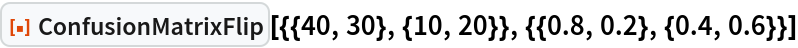Out=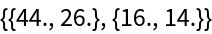Use a simplified syntax to accomplish the same flip :

 In:=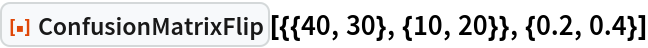Out=Flip a 3 ×3 matrix by moving 30% of those classified as 1 to 2 and 10% of those classified as 1 to 3; by moving 0% of those classified as 2 to 1 and 40% of those classified as 2 to 3; and by moving 50% of those classified as 3 to 2 and 10% of those classified as 3 to 1:

 In:=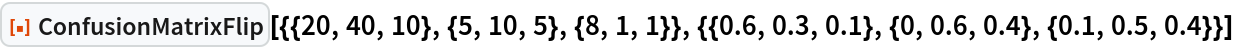Out=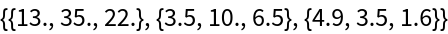### Scope (1)

Both the confusion matrix and the flip matrix can be symbolic:

 In:=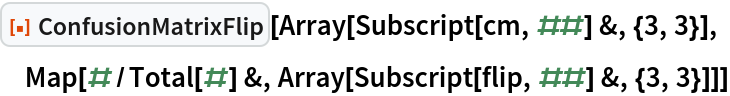Out=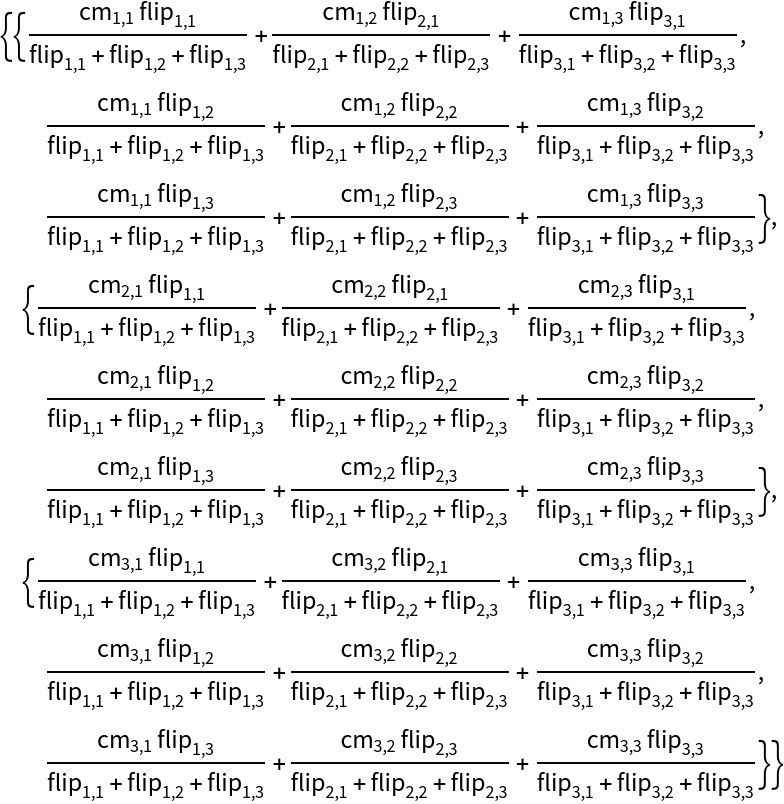### Applications (3)

Find out how much the accuracy of a classifier declines using a symbolic confusion matrix and symbolic set of flips:

 In:=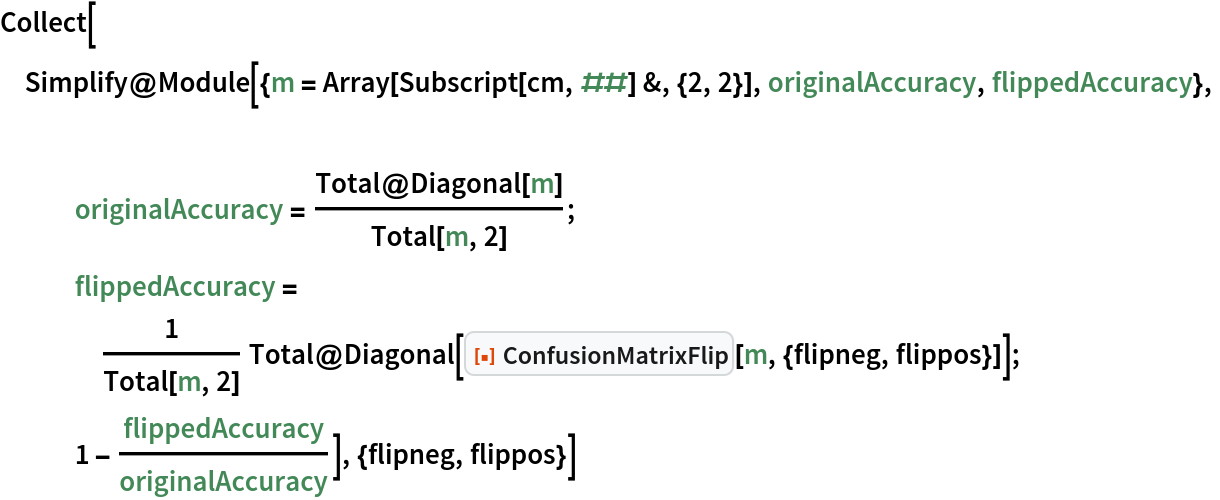Out=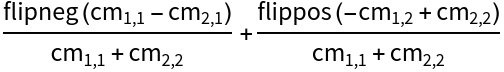Given an original 2×2 confusion matrix, find the set of flips such that the false positive rate and true positive rate equal some pair {fpr,tpr}:

 In:=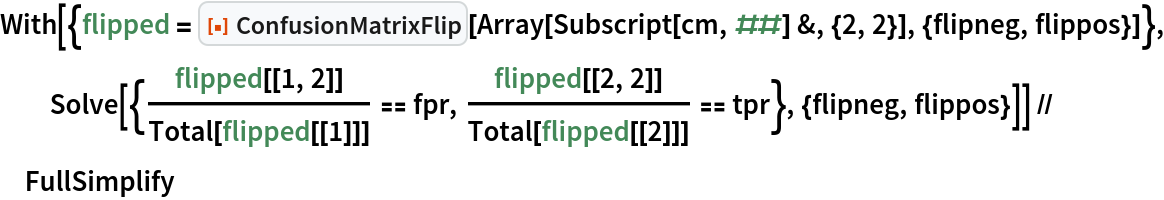Out=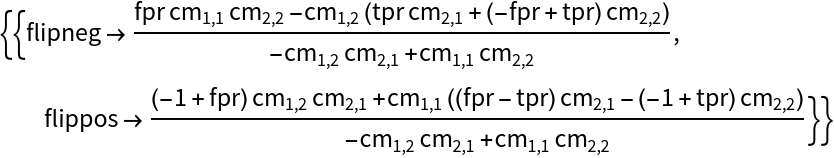Given an original 2×2 confusion matrix, find the set of flips such that the positive classification rate equals some value pcr:

 In:=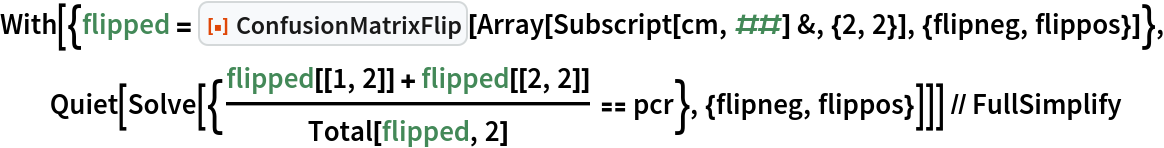Out=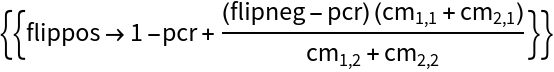### Neat Examples (2)

Show the set of possible false positive rates and true positive rates attainable by flipping a confusion matrix derived from a classifier:

 In:=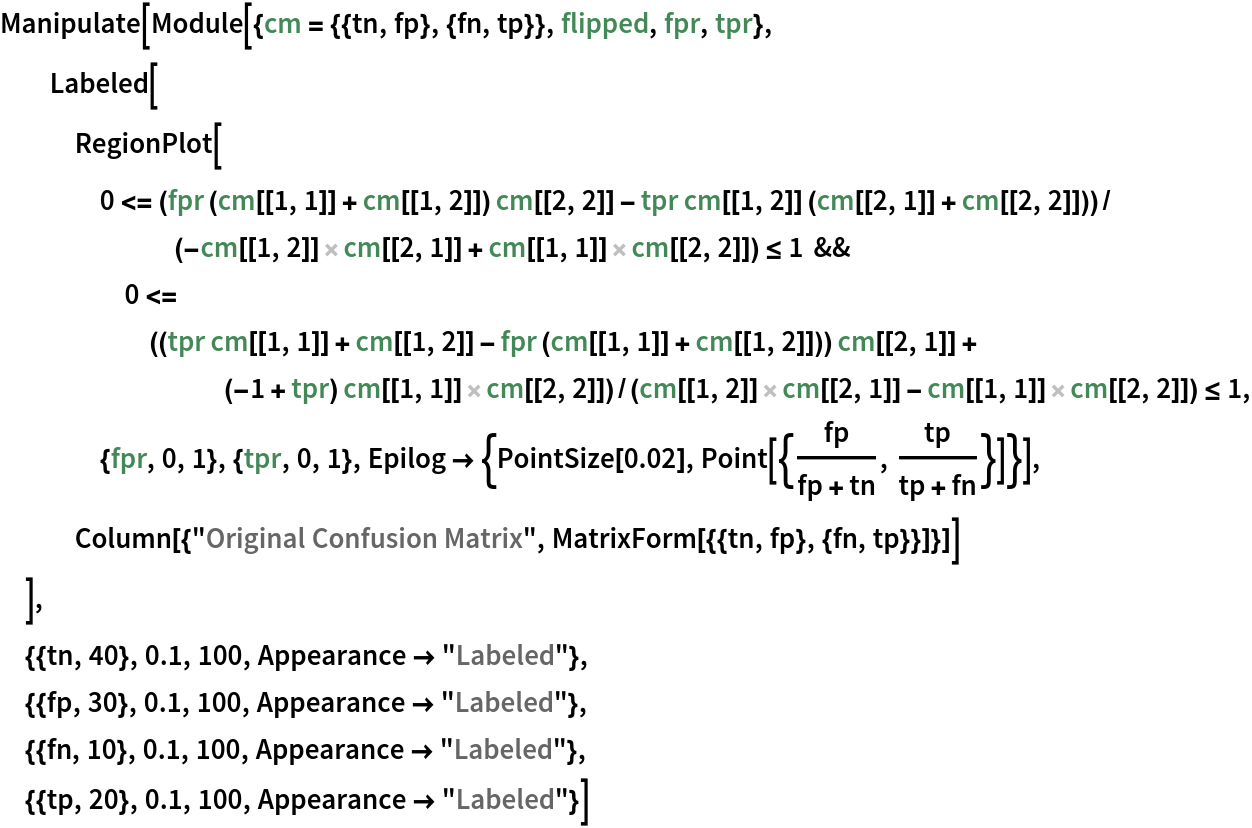Out=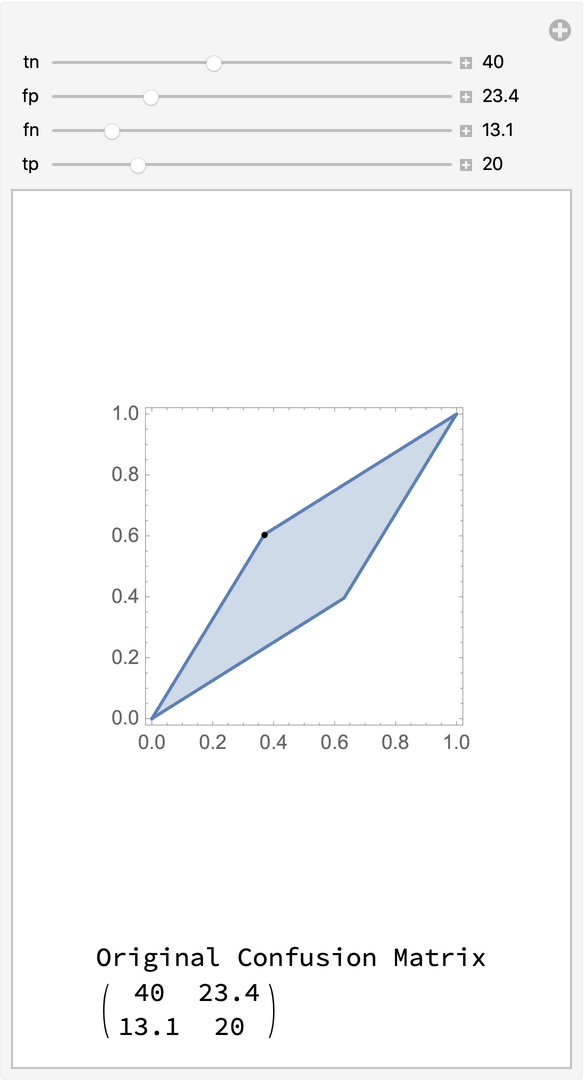Approximate the region of attainable false positive and true positive rates given a receiver operating characteristic curve (shown as a dotted line) and a set of flips:

 In:=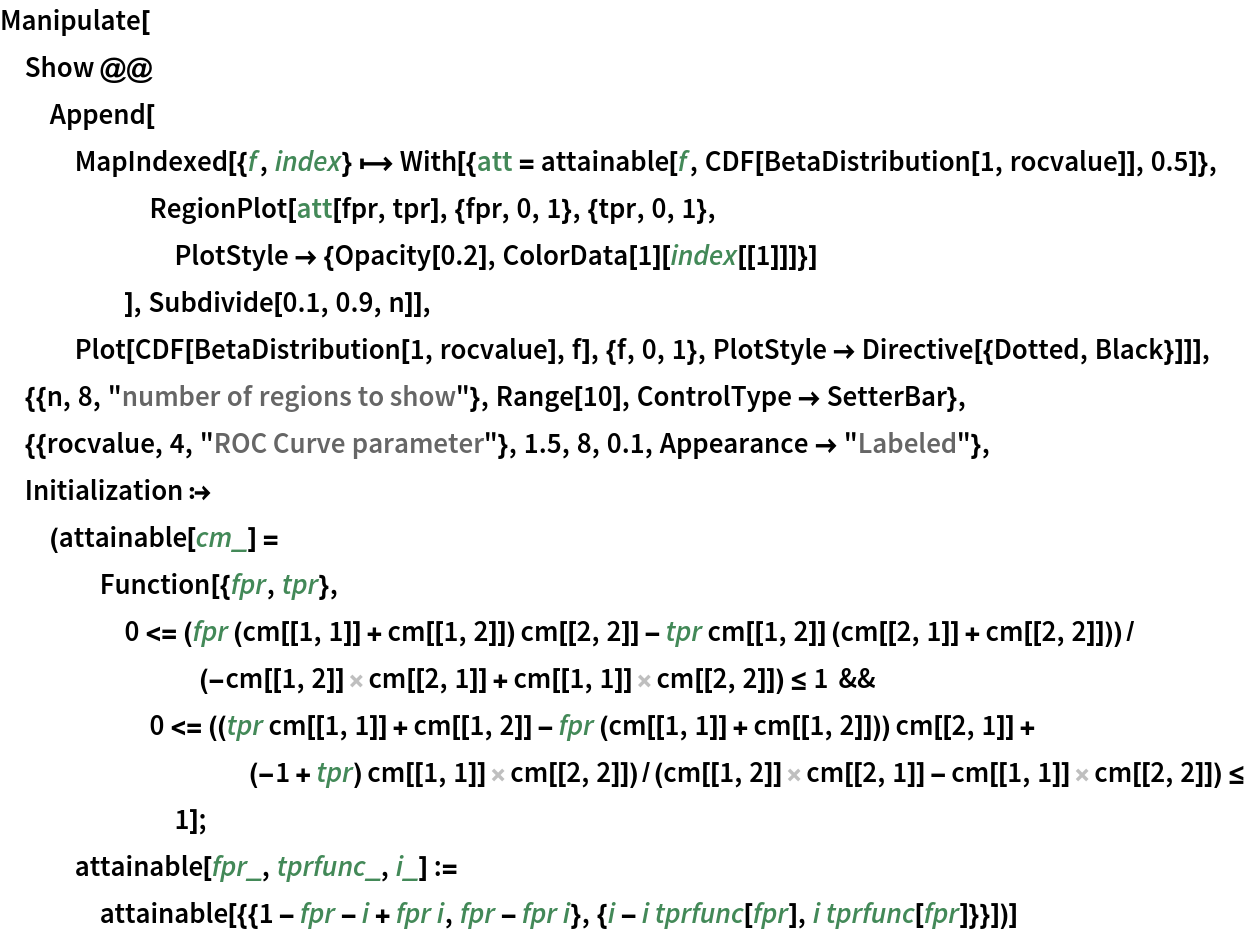Out=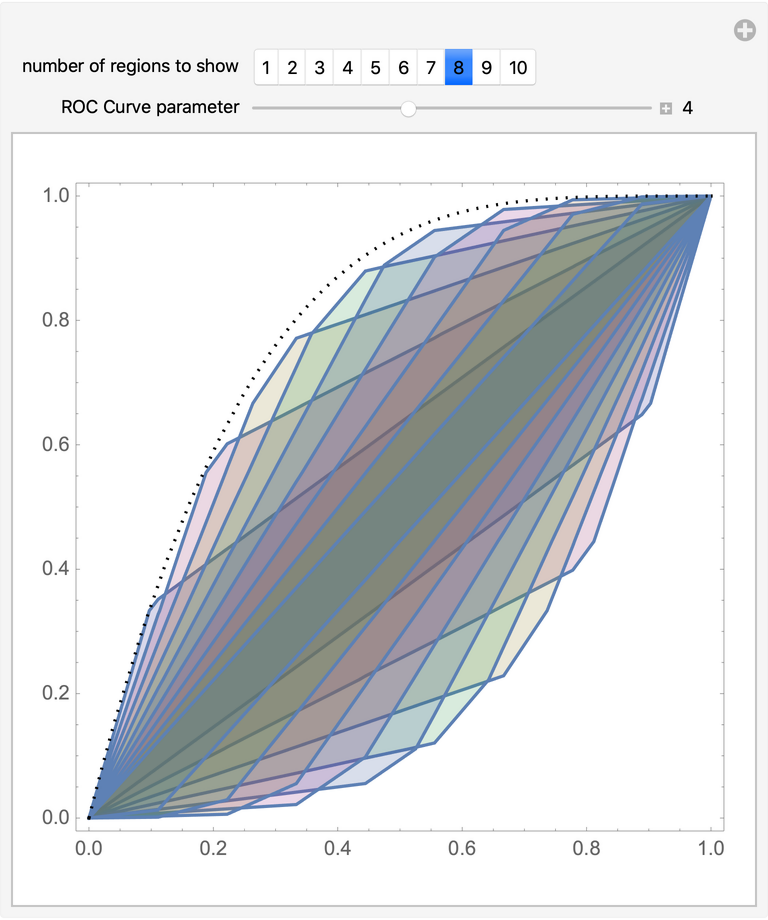Seth J. Chandler

## Version History

• 1.0.0 – 23 October 2020